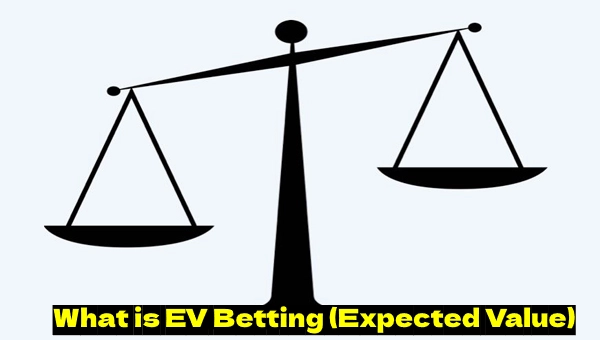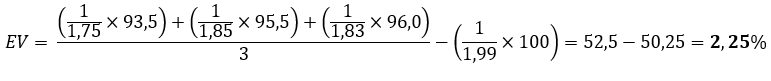Blog

# What is EV Betting (Expected Value)

Many bettors heard about the EV Betting, but not all of them understand how to apply said strategy in practice. As you may already understood, today we are going to focus on the value bets and understand their effectiveness through a real example.

Negative -EV is a situation in sports betting when the bettors's expected profit from a wager is negative in the long run. The probability of winning in the game is lower than expected.

Positive +EV, on the other hand, is a situation when the bettor's expected profit from a wager is positive in the long run. The probability of winning in the game is higher than expected. In other words, over a long period of time (100 bets), you need to find sports events where one of the outcomes has a positive expected value (+EV) and place bets on such outcomes.

## What is EV Betting?

EV betting is an effective strategy based on searching for profitable/undervalued by the bookmakers outcomes. The bettor need to search for and bet only on the outcomes having more chances of winning than estimated by the bookmakers. Value betting is not a win-win strategy, but it can bring tangible profit in the long run. Furthermore, a bettor can significantly increase the likelihood of its winnings in almost every bet combining EV betting with other systems.## Why do EV Bets Appear?

Undervalued odds don't appear out of nowhere, they can be generated due to a variety of reasons:

1. Incorrect analysis of the event by the bookmakers. Professional analysts working for the bookmakers can also make mistakes in determining the real probability of the outcome. This factor is especially actual for unpopular and uninformative championships.
2. The late reaction of bookmakers to the changes in sports event. The bookmakers do not always have time to track all changes in events, especially when it comes to live events. This allows the bettor to «catch» a suitable odds in the «slow» bookmaker.
3. Dropping odds in select outcomes. The bookmakers begin to reduce (and sometimes significantly) their odds set for the outcomes due to the lot of bets that results in the wrong estimation of the opposite outcome.
4. Bookmaker's mistakes. The banal mistake of the of bookmaker's employees can also become one of the main reasons for the EV betting. However, we do not recommend to bet on such outcomes because the bookmaker almost always finds its own mistakes and then pays for the wagered and won bets with a 1.0 odds.

## Calculating the Expected Value (Example for European Odds)

You can get and calculate Expected Values by using simple calculations, but it's useful to be able to evaluate the probability of an event on your own.

First you need to convert the odds into a probability using the following formula:

V = (1/K)*100, where V means the percentage probability and K is the bookmaker's odds.

## Outplay the bookies

Join our mailing list and learn to win a profit on EVERY event, regardless of who wins.

At this stage, you need to calculate not only the probabilities set by the bookmaker, but also your own ones. And then the value of the bet can be calculated using a simple formula:

Val = VG/VB, where VG means the bettor's expected probability, and VB is the bookmaker's one.

If the result (Val) is higher than 1, then we have a value bet that you can and should bet on.

Let's consider the calculation of the Expected Value using a real example. For our calculations, let’s take the "Ecuador wins" outcome in the 2022 World Cup group stage match between the national teams of Qatar and Ecuador. The bookmaker has set a 2.23 odds for this outcome, while we rated the event with a 1.9 odds. Let's calculate the probability of the outcome and get the value of the bet.

VB = (1/2.23)*100 = 44.84%
VG = (1/1.9)*100 = 52.63%
Val = 52.63% / 44.84% = 1.17

As you can see, the value is greater than 1, and it means that we have a value bet.

## Example of EV Calculation (American Odds)

As a simple and illustrative example, let's consider a hockey match between Philadelphia Flyers and Pittsburgh Penguins. The bookmaker has set the following odds:

 Team ODDS Philadelphia Flyers -122 Pittsburgh Penguins +110

We have \$100 for a bet. To determine which team to wager on, let's use the following algorithm:

### 1.Convert odds to probability (%) using one of the formulas:

If the bookmaker's line is negative (-122):

Bookmaker's line / (Bookmaker's line + 100) x 100%

Philadelphia Flyers: 122 / (122 + 100) x 100% = 54.95%

If the bookmaker's line is positive (+110):

100 / (Bookmaker's line + 100) x 100%

Pittsburgh Penguins: 100 / (110 + 100) x 100% = 47.62%

You may notice that 54.95% + 47.62% = 102.57%. The extra 2.57% represents the bookmaker's margin or vigorish on that particular event.

### 2.Calculate the potential profit or loss based on your wager.

For a \$100 bet on odds of -122, our total payout would be \$182, and the net profit would be \$82. If we lose, we would lose our \$100 wager.

### 3.Use the formula to calculate EV.

EV = (Winning percentage x Amount won on bet) - (Losing percentage x Amount lost on bet)

In our case, we need to substitute the following numbers:

EV = (0.5495 x \$82) - (0.4762 x \$100) = \$45.06 - \$47.62 = -\$2.56

We got a negative EV value of -\$2.56. This means that if you will constanyly bet on outcomes with such value, you will lose \$2.56 on each \$100 bet in the long run. Such bets should be avoided if you are aiming for long-term profitability.

## How to Use EV in Sports Betting?

In the foresaid example, we viewed a scenario where the bookmaker set the chance of Philadelphia Flyers winning at 54.95%. However, based on your own analysis or betting lines of ethalon bookmakers that believe the chance is actually higher, you may assume that the chance of Philadelphia Flyers winning is 60%. In that case, we would have the following EV value:

EV = (0.6 x \$82) - (0.4257 x \$100) = \$49.2 - \$42.57 = +\$6.63

This is now a positive +EV. Therefore, we can recommend to place a bet on such outcome.

The main complexity here is in determining the true probability (through analysis or by comparing different bookmakers). We recommend to pay attention on the EV Betting tool by BetBurger. With this tool, you can outsmart any bookmaker!

### Formula for determining American odds based on given probability

To determine American odds based on a probability expressed in percentages, you need to use one of the following formulas:

1.If the probability of an event is more than 50%, use the following formula:

((probability) / (100 - probability)) x 100
2.If the probability of an event is less than 50%, use the following formula:

((100 - probability) / probability) x 100

## How Can the Calculation of Expected Value be Useful in Sports Betting?

Value betting is widely used by experienced bettors due to the strategy having a number of undeniable advantages. We'll not describe all the pros of EV Betting – here are the most important of them:

• Value bets are highly effective in the long run, bringing 10-15% of the profit from the stake amount monthly (on average);
• EV betting is more profitable than surebetting, because using the strategy allows the bettor not to cover the second lever with another bet (which probably lose later on);
• Value betting allows the bettor to get tangible profits from a competent analysis of events. For example, you can find a "weak point" in the bookmaker's analysis of a certain championship and bet on its events exclusively.

## Vig and Expected Value

All bookmakers include the margin (their guaranteed profit) into its odds. The usual margin is 3-5%, but there are the bookies charging their clients up to 8-10%.

The bookmaker's margin plays a significant role in value betting. When calculating the betting probability, the bettor is not taking the margin into account, setting the equal chances of the teams as 50% to 50%. At the same time, the bookmakers will set the equal team chances as 52.5%, since the total probability must exceed 100% due to the size of the margin (in our case, we took the average margin value of 5%). So the bookmaker set the probability of an event not as 100%, but as 105%.

When searching for value bets, never account for a margin when calculating your own betting probability of the outcome of an event but also do not forget that the bookies do so. Doing so, you will be able to find more value bets, significantly increasing your chances of success.

## How to Calculate Vig on a Real Example (US Format Odds)?

Let's consider a match between Team #1 and Team #2. In this particular match, only one outcome is possible - either Team #1 wins or Team #1 loses. The bookmaker offers the following odds:

 Team ODDS TEAM 1 +120 TEAM 2 -140

1.Let's convert the bookmaker's odds of +120 into a percentage probability using the formula for positive odds (+120):

100 / (120 + 100) x 100% = 45.45%

2.Let's convert the bookmaker's odds of -140 into a percentage probability using the formula for negative odds (-140):

140 / (140 + 100) x 100% = 58.33%

3.Now let's add the probabilities together:

45.45% + 58.33% = 103.78%

Now we can determine the exact value of the vigorish (Vig): 103.78% - 100% = 3.78%. This value represents the bookmaker's margin (or Vig) embedded in this event.

You need to understand that it is not only important to find discrepancies in odds but also to ensure that the discrepancy is greater than the value of the Vig (in our case, 3.78%). If it turns out to be smaller, your bet will not be considered +EV.

## How Does Our Value Bet Scanner Work?

It is quite time-consuming to search for value bets on your own - you need to manually evaluate the events and make all necessary calculations. However, in the world of betting, there are value bet scanners which make the process as easy as possible. Among the truly handy scanners, can't be ignored the BetBurger service.

Our value bet scanner takes over the entire process of value bet scanning, offering you all suitable events in a matter of seconds. All you have to do then is wager your bet and wait for the result. BetBurger software analyzes data from over 200 bookmakers across 40+ of sports. With it, you can always easily and quickly find value bets using the EV betting strategy.

## Example of Finding +EV Bets Using the BetBurger Scanner

 Bookmakers ODDS Payout B1 for calculation 1,75 93,5\$ B2 for calculation 1,85 95,5\$ B3 for calculation 1,83 96,0\$ B1 for display 1,99 100\$

B1 for display (Valuebet) is a bookmaker that searches for Valuebets (inflated odds).

B1/B2/B3 for calculation refers to the bookmaker against which the search for favorable odds is conducted.

Payout is the total return amount for bets on opposing outcomes, totaling 100.

You should understand that the higher the bookmaker's margin, the lower the payout. According to our formula, the payout for the Valuebet display will be 100\$.Based on the calculation formula, it can be seen that the odds of 1.99 are inflated by the bookmaker by 2.25%. We can gain an advantage of 2.25% from the bookmaker because their line has an error. We determined this by comparing the odds for a specific outcome in our bookmaker and several top bookmakers.

## Used literature, references

You may also likeArtur Polianskyi
16
Jun 10, 2022Artur Polianskyi
1
May 23, 2022Artur Polianskyi
60
Apr 15, 2022